SEARCH HOMEMath Central Quandaries & QueriesQuestion from Clint: I am stuck on a puzzle for Geocaching which is a GPS game. I know it is a number matrix but don't know how to start. [1 -7 16 -3 -9; 1 -3 18 -20 -33] x [1 8 -9 3 5; 0 1 0 4 2; 0 0 1 2 1; 0 0 0 1 -1; 0 0 0 0 1] = ? Many thanks any help will be greatly appreciated.Hi Clint.

I drew out your matrix question in a more standard way: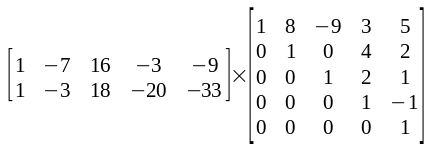You can refer to http://en.wikipedia.org/wiki/Matrix_multiplication for the full understanding of Matrix multiplication, but in a nutshell, when you multiply an 2 by 5 matrix times a 5 by 5 matrix, the result is 2 by 5.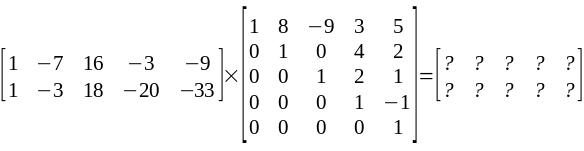For each cell in the result, you calculate by adding the products of the first matrix's row with the second matrix's column.

Here's an example: Say I want to find the value of the green cell -- that's cell location (2, 4) because I count from (1, 1) in the upper left corner.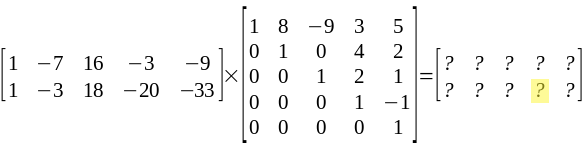To do this, I concentrate on ROW 2 from the first matrix and COLUMN 4 from the second: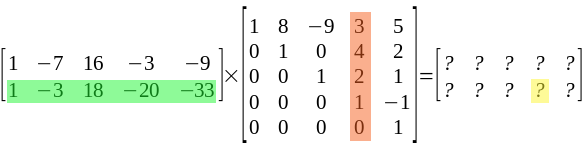Now I multiply the values in the first cells together: 1 x 3 = 3.
Now the second values: -3 x 4 = -12
And the third: 18 x 2 = 36
The fourth: -20 x 1 = -20
The fifth: -33 x 0 = 0
Finally, I add up these products: 3 + (-12) + 36 + (-20) + 0 = 7. So the result here is a 7.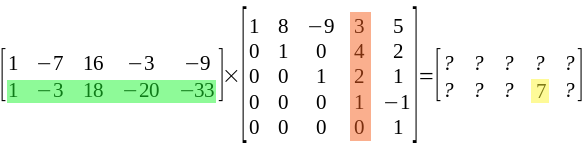I leave the rest of the work up to you!! Hope you find what you're geocaching for!

Cheers,
Stephen La RocqueMath Central is supported by the University of Regina and The Pacific Institute for the Mathematical Sciences.